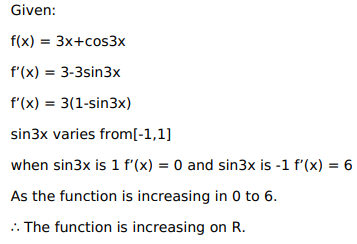# Solve this following

Question:

Mark $(\sqrt{)}$ against the correct answer in the following:

The function $f(x)=3 x+\cos 3 x$ is

A. increasing on $R$

B. decreasing on $\mathrm{R}$

C. strictly increasing on $R$

D. strictly decreasing on $R$

Solution: